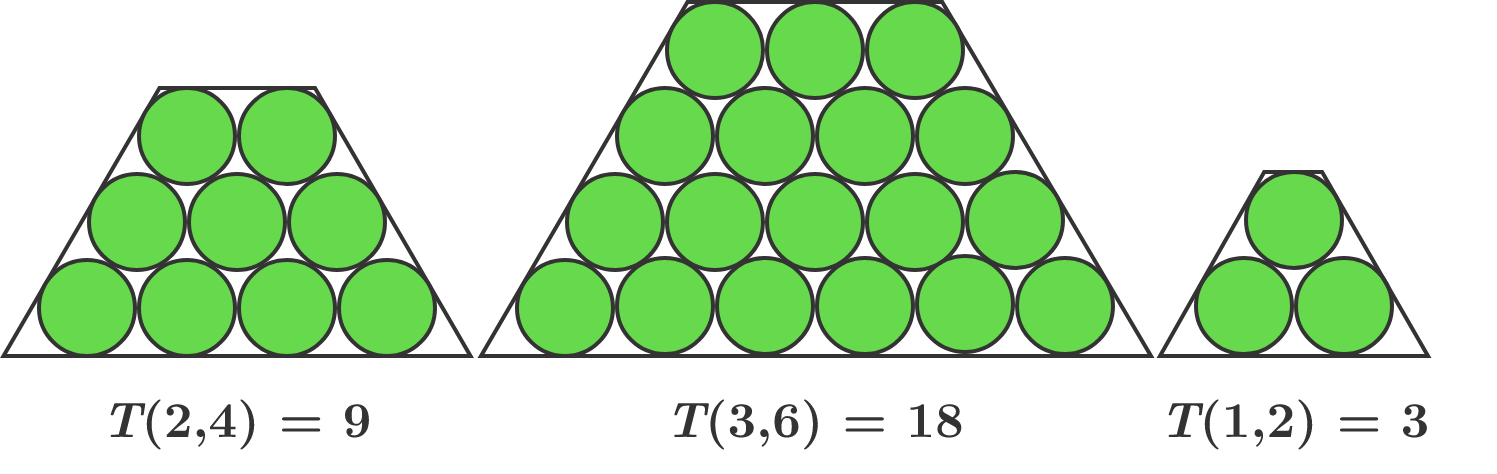# Trapezoidal Numbers

$T(x, y)$ is the number of circles in a trapezoidal arrangement such that $x$ is the number of circles in the top row and $y$ is the number of circles in the bottom row. Each row has one fewer circle than the row beneath it does.What is the only number between 10000 and 20000 that cannot be a value of $T(x, y)$ for positive integers $x$ and $y$ such that $x < y?$

×